### Home > CALC > Chapter 11 > Lesson 11.3.1 > Problem11-97

11-97.
1. Examine the integrals below. Consider the multiple tools available for integrating and use the best strategy. After evaluating each integral, write a short description of your method. Homework Help ✎

1.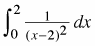2.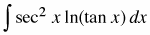3.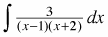4.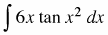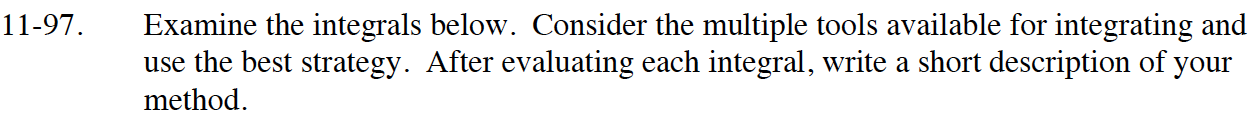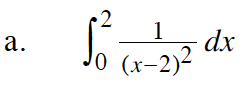The Reverse Chain Rule can be used here, but this is an improper integral, so be sure to use a limit.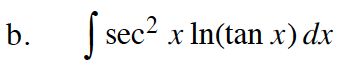Let u = tan(x).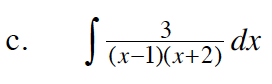Use partial fraction decomposition.

$\frac{A}{x-1}+\frac{B}{x+2}=\frac{3}{(x-1)(x+2)}$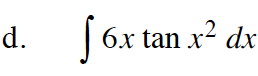Use substitution twice.

Let u = x2. Then du = 2x.

$= \int 3\tan(u)du$

Let v = cos(u). Then dv = –sin(u)du.

$=-3\int\frac{1}{v}dv$